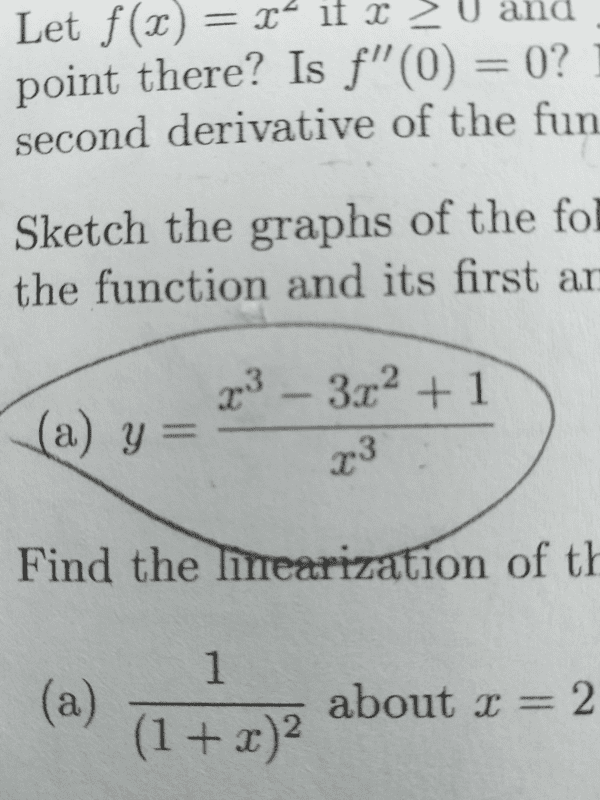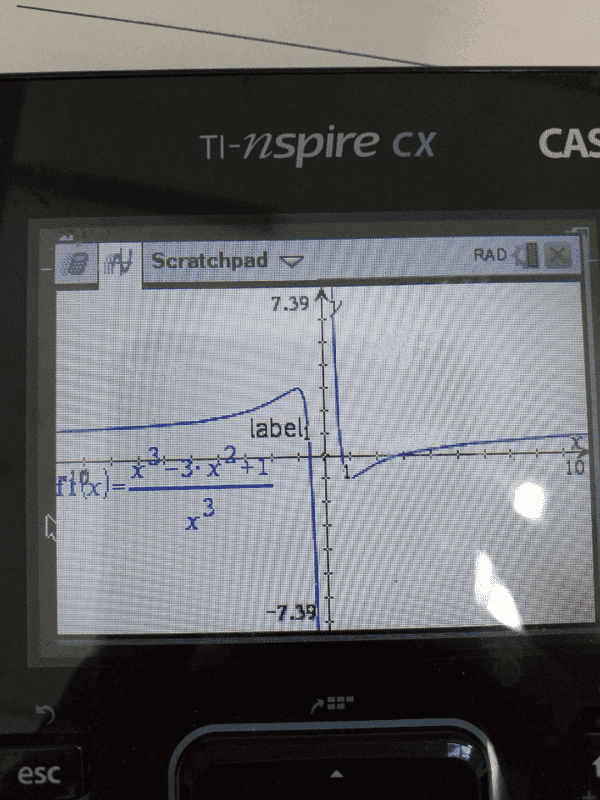# Question about asymptotes of rational function

Sunwoo Bae
Homework Statement:
sketch the graph of (x^3-3x^2+1)/x^3, making use of any suitable information you can obtain from the function and its first and second deerivative
Relevant Equations:
If both polynomials are the same degree, divide the coefficients of the highest degree termsI tried graphing the function in the calculator, and the graph seems to have a horizontal asymptote at y=0, not at y=1. Why is this so?

Thanks for helping out.

Staff Emeritus
Homework Helper
Gold Member
Homework Statement:: sketch the graph of (x^3-3x^2+1)/x^3, making use of any suitable information you can obtain from the function and its first and second derivative
Relevant Equations:: If both polynomials are the same degree, divide the coefficients of the highest degree terms

View attachment 263848
View attachment 263849

I tried graphing the function in the calculator, and the graph seems to have a horizontal asymptote at y=0, not at y=1. Why is this so?

Thanks for helping out.
That graph looks consistent with having a horizontal asymptote of ##y=1## .

You were given the hint to divide the coefficients of the highest degree terms. That gives ##1## , which indicates that there is a horizontal asymptote: ##y=1## .

Better than that is to express the function as follows.

##y=\dfrac{x^3}{x^3} + \dfrac{-3x^2+1}{x^3}##

##y=1 + \dfrac{-3x^2+1}{x^3}##

You may be able to see from this, what the horizontal asymptote is. Also, using this expression, it may be easier to get the derivatives (1st & 2nd) as asked for.

Last edited:
•scottdave
archaic
You get the horizontal asymptotes by seeing if the function has any limits as ##x\to\pm\infty##.
I think that what you meant is that there's vertical asymptote at ##x=0##.

•SammyS
Mentor
I think that what you meant is that there's vertical asymptote at ##x=0##
It's pretty clear to me that he meant exactly what he said.
I tried graphing the function in the calculator, and the graph seems to have a horizontal asymptote at y=0, not at y=1.

•SammyS and archaic
MidgetDwarf
For rational functions, to check for horizontal asymptotes (the behavior of the function when x gets very large in negative x and positive x direction), we divide every term by the highest power of x that appears. Then we take the limit as x approaches -infinity and the limit as x approaches + infinity.

I am assuming this is for Pre-Calculus. So if you were to sketch these types of functions, using stuff you learned (including what I am stating in the paragraph), then your graph would not be as accurate as what appears on the graphing calculator. Moreover, would be time consuming to do so the more complicated the function.